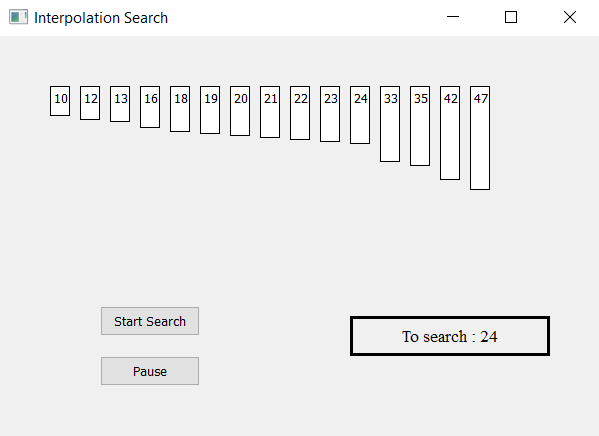Related Articles

# Interpolation Search visualizer using PyQt5

• Last Updated : 29 May, 2021

In this article we will see how we can make a PyQt5 application which will visualize the interpolation search algorithm.
The Interpolation Search is an improvement over Binary Search for instances, where the values in a sorted array are uniformly distributed. Binary Search always goes to the middle element to check. On the other hand, interpolation search may go to different locations according to the value of the key being searched. For example, if the value of the key is closer to the last element, the interpolation search is likely to start search toward the end side.GUI implementation steps :
1. Create a list of label according to the given list of numbers
2. Set their text, border, color and geometry with a respective gap from each other
3. Each label height should be proportional to the value of each number
4. Create a start and pause push button to start the searching and pause the searching
5. Create a result label to show the searching status
Back end implementation steps :
1. Create label list corresponding to the given numbers
2. Create variable for the lower higher and position index and flag for searching
3. Add action to the push button their action should change the flag status i.e start action should make flag true and pause action should make flag false.
4. Create timer object which calls a method after every specific time
5. Inside the timer method check for the flag is flag is true begin the interpolation search algorithm
6. Check if value lies within the range if not show output as not found else continue
7. If the index of lower index is greater then higher index stop the search and show result as not found
8. Calculate the position index through the interpolation search formula
9. Check if the desired value is at position index if matches show result as found
10. If desired value is not equal then update the lower and higher index according to the desired value.

Below is the implementation

## Python3

 `# importing libraries``from` `PyQt5.QtWidgets ``import` `*``from` `PyQt5 ``import` `QtCore, QtGui``from` `PyQt5.QtGui ``import` `*``from` `PyQt5.QtCore ``import` `*``import` `sys`  `class` `Window(QMainWindow):` `    ``# list of numbers``    ``number ``=` `[``10``, ``12``, ``13``, ``16``, ``18``, ``19``, ``20``, ``21``,``                  ``22``, ``23``, ``24``, ``33``, ``35``, ``42``, ``47``]` `    ``# desired value``    ``desired ``=` `24``    ``def` `__init__(``self``):``        ``super``().__init__()` `        ``# setting title``        ``self``.setWindowTitle(``"Interpolation Search "``)` `        ``# setting geometry``        ``self``.setGeometry(``100``, ``100``, ``600``, ``400``)` `        ``# calling method``        ``self``.UiComponents()` `        ``# showing all the widgets``        ``self``.show()` `    ``# method for widgets``    ``def` `UiComponents(``self``):` `        ``# start flag``        ``self``.start ``=` `False` `        ``# list to hold labels``        ``self``.label_list ``=` `[]` `        ``# position for keeping index``        ``self``.position ``=` `0` `        ``# lower index``        ``self``.lo ``=` `0` `        ``# higher index``        ``self``.hi ``=` `len``(``self``.number) ``-` `1` `        ``# local counter``        ``c ``=` `0` `        ``# iterating list of numbers``        ``for` `i ``in` `self``.number:` `            ``# creating label for each number``            ``label ``=` `QLabel(``str``(i), ``self``)` `            ``# adding background color and border``            ``label.setStyleSheet("border : ``1px` `solid black;``                                 ``background : white;")` `            ``# aligning the text``            ``label.setAlignment(Qt.AlignTop)` `            ``# setting geometry using local counter``            ``# first parameter is distance from left``            ``# and second is distance from top``            ``# third is width and forth is height``            ``label.setGeometry(``50` `+` `c ``*` `30``, ``50``, ``20``, i ``*` `2` `+` `10``)` `            ``# adding label to the label list``            ``self``.label_list.append(label)` `            ``# incrementing local counter``            ``c ``=` `c ``+` `1`  `        ``# creating push button to start the search``        ``self``.search_button ``=` `QPushButton(``"Start Search"``, ``self``)` `        ``# setting geometry of the button``        ``self``.search_button.setGeometry(``100``, ``270``, ``100``, ``30``)` `        ``# adding action to the search button``        ``self``.search_button.clicked.connect(``self``.search_action)` `        ``# creating push button to pause the search``        ``pause_button ``=` `QPushButton(``"Pause"``, ``self``)` `        ``# setting geometry of the button``        ``pause_button.setGeometry(``100``, ``320``, ``100``, ``30``)` `        ``# adding action to the search button``        ``pause_button.clicked.connect(``self``.pause_action)` `        ``# creating label to show the result``        ``self``.result ``=` `QLabel(``"To search : "` `+` `str``(``self``.desired), ``self``)` `        ``# setting geometry``        ``self``.result.setGeometry(``350``, ``280``, ``200``, ``40``)` `        ``# setting style sheet``        ``self``.result.setStyleSheet(``"border : 3px solid black;"``)` `        ``# adding font``        ``self``.result.setFont(QFont(``'Times'``, ``10``))` `        ``# setting alignment``        ``self``.result.setAlignment(Qt.AlignCenter)` `        ``# creating a timer object``        ``timer ``=` `QTimer(``self``)` `        ``# adding action to timer``        ``timer.timeout.connect(``self``.showTime)` `        ``# update the timer every 200 millisecond``        ``timer.start(``200``)` `    ``# method called by timer``    ``def` `showTime(``self``):` `        ``# checking if flag is true``        ``if` `self``.start:` `            ``# Interpolation Search``            ``# checking if desired number is with in the range``            ``if` `(``self``.desired < ``self``.number[``self``.lo] ``or``                  ``self``.desired > ``self``.number[``self``.hi]):` `                ``# make flag false``                ``self``.start ``=` `False``                ``# show result as not found``                ``self``.result.setText(``"Not Found"``)` `            ``# if lower index become greater then higher index``            ``if` `self``.lo > ``self``.hi:` `                ``# make flag false``                ``self``.start ``=` `False``                ``# show result as not found``                ``self``.result.setText(``"Not Found"``)` `            ``else``:``                ``# lower index is equal to higher index``                ``if` `self``.lo ``=``=` `self``.hi:` `                    ``# checking if it matches desired value``                    ``if` `self``.number[``self``.lo] ``=``=` `self``.desired:` `                        ``# show result``                        ``self``.result.setText(``"Found at index : "` `+` `str``(``self``.lo))``                        ``# make result label color green``                        ``self``.label_list[``self``.lo].setStyleSheet(``                                     ``"border : 2px solid green;"``                                     ``"background-color : lightgreen;"``)` `                    ``else``:``                        ``# make result color grey``                        ``self``.label_list[``self``.lo].setStyleSheet(``                                     ``"border : 1px solid black;"``                                     ``"background-color : grey;"``)` `                ``# Probing the position with keeping``                ``# uniform distribution in mind.``                ``self``.position ``=` `self``.lo ``+` `int``(((``float``(``self``.hi ``-` `self``.lo) ``/``                        ``( ``self``.number[``self``.hi] ``-` `self``.number[``self``.lo])) ``*``                          ``(``self``.desired ``-` `self``.number[``self``.lo])))` `                ``# checking if the position matches with desired value position``                ``if` `self``.number[``self``.position] ``=``=` `self``.desired:` `                    ``# make flag false``                    ``self``.start ``=` `False` `                    ``# show result and make label color green``                    ``self``.result.setText(``"Found at position : "` `+` `str``(``self``.position))``                    ``self``.label_list[``self``.position].setStyleSheet(``                                        ``"border : 2px solid green;"``                                        ``"background-color : lightgreen;"``)` `                ``else``:` `                    ``# make label color grey``                    ``self``.label_list[``self``.position].setStyleSheet(``                                      ``"border : 1px solid black;"``                                      ``"background-color : grey;"``)` `                ``# If desired is larger, desired is in upper part``                ``if` `self``.number[``self``.position] < ``self``.desired:``                    ``# updating lowe index``                    ``self``.lo ``=` `self``.position ``+` `1` `                ``# If desired is smaller, desired is in lower part``                ``else``:``                    ``# updating higher index``                    ``self``.hi ``=` `self``.position ``-` `1`   `    ``# method called by search button``    ``def` `search_action(``self``):` `        ``# making flag true``        ``self``.start ``=` `True` `        ``# showing text in result label``        ``self``.result.setText(``"Started searching..."``)` `    ``# method called by pause button``    ``def` `pause_action(``self``):` `        ``# making flag false``        ``self``.start ``=` `False` `        ``# showing text in result label``        ``self``.result.setText(``"Paused"``)`  `# create pyqt5 app``App ``=` `QApplication(sys.argv)` `# create the instance of our Window``window ``=` `Window()` `# start the app``sys.exit(App.``exec``())`

Output :

Attention geek! Strengthen your foundations with the Python Programming Foundation Course and learn the basics.

To begin with, your interview preparations Enhance your Data Structures concepts with the Python DS Course. And to begin with your Machine Learning Journey, join the Machine Learning – Basic Level Course

My Personal Notes arrow_drop_up